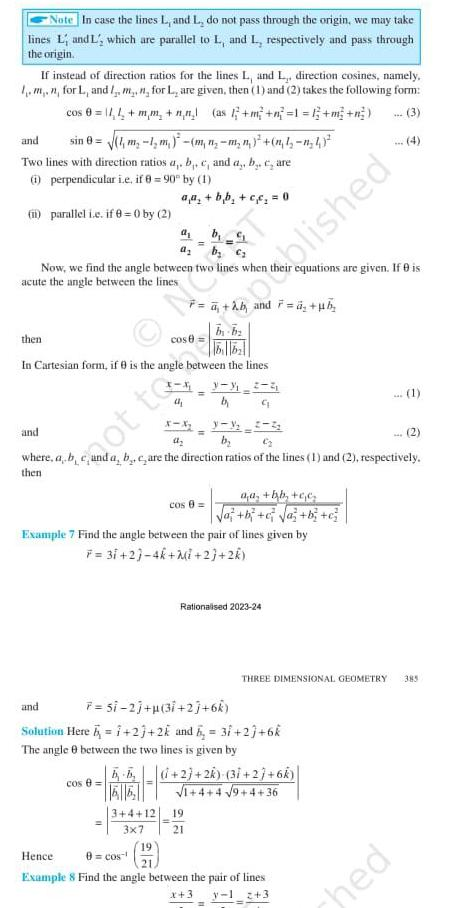Question:

# Note In case the lines L and L do not pass through the

Last updated: 9/16/2023Note In case the lines L and L do not pass through the origin we may take lines L and L which are parallel to L and L respectively and pass through the origin If instead of direction ratios for the lines L and L direction cosines namely 1 m n for L and l m n for L are given then 1 and 2 takes the following form cos 0 1 mm nn as m 1 m n and sin 0 1 m 1 m m n m n n 1 1 4 Two lines with direction ratios a b c and a b c are i perpendicular i e if 0 90 by 1 ii parallel i e if 0 0 by 2 a a by C Now we find the angle between two lines when t acute the angle between the lines then aa bb ce 0 F a Ab and F b b b B In Cartesian form if 0 is the angle between the lines 3 3 2 2 b cos8 4 not to cos 8 19 T M cos 8 9 56 3 4 12 19 3x7 21 X X y 2 2 and a by where a b c und a b c are the direction ratios of the lines 1 and 2 respectively then Example 7 Find the angle between the pair of lines given by F 3i 2 4k M 2 2k aa bb cc b b c Rationalised 2023 24 and 7 57 2j 37 2j 6k Solution Here 7 23 2k and h 3i 2 6k The angle 8 between the two lines is given by bb 2 2k 37 27 6k THREE DIMENSIONAL GEOMETRY equations are given If 0 is 1 4 4 9 4 36 3 Hence 0 cos Example 8 Find the angle between the pair of lines x 3 y 1 2 3 published 4 ched 1 2 385